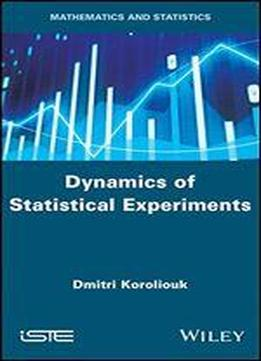# Dynamics Of Statistical Experiments by Dmitri Koroliouk / 2020 / English / PDF

This book is devoted to the system analysis of statistical experiments, determined by the averaged sums of sampling random variables. The dynamics of statistical experiments are given by difference stochastic equations with a speci?ed regression function of increments linear or nonlinear. The statistical experiments are studied by the sample volume increasing (N ??), as well as in discrete-continuous time by the number of stages increasing (k ??) for different conditions imposed on the regression function of increments. The proofs of limit theorems employ modern methods for the operator and martingale characterization of Markov processes, including singular perturbation methods. Furthermore, they justify the representation of a stationary Gaussian statistical experiment with the Markov property, as a stochastic difference equation solution, applying the theorem of normal correlation. The statistical hypotheses verification problem is formulated in the classification of evolutionary processes, which determine the dynamics of the predictable component. The method of stochastic approximation is used for classifying statistical experiments.

views: 377